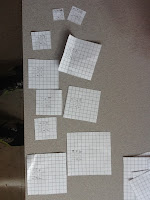## Monday, April 29, 2013

### 26 squaresAll the squares of side lengths 1 to 26
In looking for an activity to demonstrate "Pythagorean theorem", a colleague and I decided to cut out all the squares of side length 1 to 26 like this. Actually we had the students do it on the first day of class. Once they had them cut out we asked them to make something - we got robots, wedding cakes, smiley faces, guns :( , you name it they made it. Then we asked students to make something with 3 of the squares. Eventually one of them made a triangle with three of the squares. The sides of the 3 squares making the sides of the triangle.

Once we decided to explore these triangles made from the hollow of the squares, this led to a nice discussion about when we would get a triangle or when it was impossible. We generated a list of side lengths of the 3 squares that would create a triangle ( for example 12, 15 and 20 ) and a list of side lengths of the 3 squares that would not create a triangle ( for example 3, 5 and 17 ).
Triangle inequality: The sum of the lengths of the two smaller sides must be greater than the longest side in a triangle.

After this discussion we talked about different classifications of triangles based on angles. So we then generated a list of side lengths that would make an acute triangle ( for example 19, 20 and 21 ), an obtuse triangle ( for example 5, 11 and 15 ) and last but not least the hunt for a right angled triangle. Once we established the rule for right triangles: the area of the two smaller squares must equal the area of the largest square the students were on the hunt for all possible triangles using the squares with side lengths 1 to 26. This is what the board looked like by the end of the period.

There were 4 families: FAMILY 1, 3, 4, and 5 and all it's multiples (6, 8, 10; 9, 12, 15; 12, 16, 20; 15, 20, 25), FAMILY 2, 5, 12, 13 and it's multiple (10, 24, 26), FAMILY 3, 8, 15, 17 and FAMILY 4, 7, 24, 25. Nine sum of squares triples amongst 4 families. The students had a fun search for these. We consolidated on how to find an unknown side in a right triangle.

We spent three days doing all this-time well spent.

Next we took the 3, 4, 5 family and explored the relationship between the side lengths amongst the different triangles. Students made connections about the triangles being scaled by some factor. They also decided that the angles in these triangles would be exactly the same. We called these families of triangles similar triangles. With a day of consolidation we spent two days on similar triangles.

Of course I wanted them to tell me what the angles in a 3, 4, 5 triangle were  (or any of the triangles in that family). They decided the only angle they knew was the 90 degree angle and the other two would have to be measured with a protractor. After measuring all the angles in a couple of different triangles in the family the students decided on the smallest angle being 37 degrees and the middle angle being 53 degrees.

I then pulled a trigonometric table and explained the words opposite, adjacent and hypotenuse and we checked out the ratios for a 3, 4 and 5 triangle. Of course the students reaction was who would make this sort of table. We worked through some examples where they found an angle, and some where they found a side length. Trig table only at this point. Again with a couple of days of consolidating we spent three days on this.

Next we made three representations ( table, graph, equation ) for side length of the squares and perimeter of the squares. P=4L. Linear relations and their properties and solving linear equations. Students spent some time with a graphing calculator and learned how to do a linear regression.

Again three representations ( table, graph, equation ) for side length of the square and area of the squares. A=L^2. Quadratic relations and their characteristics. Students spent some time with a graphing calculator and learned how to do a quadratic regression.

The goods are here:
The Goods

Time well spent - took 3 weeks and uncovered 6 of 9 big ideas in MFM 2P math and introduced 3 out of 3 strands. A great activity to start the course  - a little dry and very scaffolded - but a nice start to the course.